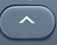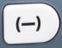# PowerBasics

Number
Power

The term power refers to how many times a number is multiplied by itself.

A power is also known as an exponent.

7 to the power of 2 = 7² = 7 × 7 = 49
7 to the power of 2 is also known as 7 squared.

7 to the power of 3 = 7³ = 7 × 7 × 7 = 343
7 to the power of 3 is also known as 7 cubed.

7 to the power of 4 = 74 = 7 × 7 × 7 × 7 = 2401

In order to get the correct answer when calculating the power of a negative number, place the negative number in parentheses to protect the order of operations.
Right:  -7 to the power of 4 = (-7)4 = 2401
Wrong:  -7 to the power of 4 = -74 = -2401

7 to the power of -4 = 7-4 =$\bf\displaystyle\frac{1}{7}$ ×$\bf\displaystyle\frac{1}{7}$ ×$\bf\displaystyle\frac{1}{7}$ ×$\bf\displaystyle\frac{1}{7}$ = 0.000416493

NumberA × Number= NumberA+B
74 × 75 = 74+5 = 79$\bf\displaystyle\frac{{{Number}^{A}}}{{{Number}^{B}}}={{Number}^{A-B}}$$\bf\displaystyle\frac{{{7}^{12}}}{{{7}^{3}}}={{7}^{12-3}}={{7}^{9}}$is the power command.

It is possible that questions involving powers will be in the calculator-prohibited section of the test.

Question

What is 96?

531441

Input Display Commentblinker clears screen6 96 9 to the power of 6531441 Answer
###### Power

Question
According to the calculator, what is the value of -96?
According to the calculator, what is the value of (-9)6
?
Observe how parentheses change the results of these calculations.

-96 = -531441

(-9)6 = 531441

The second calculation illustrates the way parentheses protect the order of operations when working with the power of a negative number.

Input Display Commentblinker clears screen96 -96-531441blinker clears screen96 (-9)6
###### Parentheses protect order of operations.531441
###### Power

Question

What is 9-6?

0.000001882

Input Display Commentblinker clears screen-6 9-6 9 to the power of -60.000001882 Answer
###### Power

Question
Without using the calculator, what is 84 × 8?  Give your answer in exponent form.

810

NumberA × Number= NumberA+B
84 × 86 = 84+6 = 810

Question
Without using the calculator, what is$\bf\displaystyle\frac{{{4}^{10}}}{{{4}^{3}}}$ ?  Give your answer in exponent form.$\bf\displaystyle{{4}^{7}}$$\bf\displaystyle\frac{{{Number}^{A}}}{{{Number}^{B}}}={{Number}^{A-B}}$$\bf\displaystyle\frac{{{4}^{10}}}{{{4}^{3}}}={{4}^{10-3}}={{4}^{7}}$

Practice – Questions
1.  What is 64?

2.  What is 6 to the power of 4?

3.  What is (-6)4?

4.  What is -6 to the power of 4?

5.  What is -64?

6.  What is 6-4?

7.  Without using the calculator, what is 37 × 3?  Give your answer in exponent form.

8.  Without using the calculator, what is 37 × 3-3 ?  Give your answer in exponent form.

9. Without using the calculator, what is$\bf\displaystyle\frac{{{5}^{12}}}{{{5}^{8}}}$ ?  Give your answer in exponent form.

10. Without using the calculator, what is$\bf\displaystyle\frac{{{5}^{12}}}{{{5}^{20}}}$ ?  Give your answer in exponent form.

1.  1296

2.  1296

3.  1296

4.  1296

5.  -1296

6.  0.000771605

7.  315

8.  34

9.  54

10.  5-8